## Mathematics Paper 2 Questions and Answers - Bunamfan Post Mock 2021 Exams

Instructions to Candidates

1. This paper contains two sections, Section I and Section II.
2. Answer all the questions in section I and any five questions in section II.
3. All the questions in section II carry equal marks.
4. Negligence and slovenly work will be penalized.
5. Mathematical tables and non-programmable electronic calculators maybe used.## QUESTIONS

SECTION I (50 Mks)
(Answer all questions in this section)

1. Use logarithms tables to evaluate. (4 marks)
2. A trader mixes grade A coffee costing sh 600 per kg, with grade B coffee costing sh. 280 per kg in the ratio 3 : 5.Find the price at which he must sell 1 kg of the mixture to make a profit of 20 % . (4 marks)
3. Given that cos θ =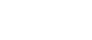, find the value of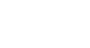in its simplest form. (Leave your answer in surd form) (3 marks)
4. Determine the equation of the normal to the curve y = 3x2 - 4x + 5 at the point (1, 4). (3 marks)
5. Water flows from a pipe at the rate of 250 litres per minute. If the pipe is used to drain a tank full of water measuring 3.2m by 2.5m by 2m, how long would it take to drain the tank completely when it is 3/4 full? (3 marks)
6. Make N the subject of the formula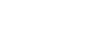(2 marks)
7. Determine the period and amplitude of the function. y = 4 sin (2x - 20°) (2 marks)
8. In the figure below, PA is 1.2cm shorter than PD. Given that AB = 15.6cm, CD = 9.6cm,Determine the length of PA. (3 marks)
9. Without using logarithms table or calculator, solve for x in;
log 5 – 2 + log(2x + 10) = log (x - 4) (3 marks)
10. In an arithmetic progression, the 20th term is 92 and the sum of the first 20 terms is 890. Calculate;
1. The first term (2 marks)
2. The common difference (1 mark)
11. Solve for θ in the equation sin (3θ + 120º) =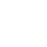for 0 ≤ θ ≤ 180º. (3 marks)
12.
1. Expand and simplify the expression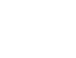up to the third term. (2 marks)
2. Hence use the expansion in (a) above to approximate the value of (39.6)5 (2 marks)
13. The cost per head for catering for a party is partly constant and partly varies inversely as the number of people expected. The cost per head for a party of 100 people is Sh. 1860 and that for 180 people is sh. 1060. Find the cost per head for 200 people. (3 marks)
14. A body is moving along a straight line and its acceleration after t seconds is (5 – 2t) ms-2. Its initial velocity Vms-1 is 4ms-1. Find V in terms of t. (3 marks)
15. Determine the turning points for the curve y = 5x – 8x2 + x3. (4 marks)
16. Draw a line PQ = 7.2cm and on one side of the line, use a ruler and pair of compasses only to draw the locus of a point A such that ∠ PAQ = 60º and on it mark point A such that PA = QA (3 marks)

SECTION II (50 Mks)
(Answer any FIVE questions from this section)

1. The table below represents marks scored in a mathematics test.
 Marks 10-19 20-29 30-39 40-49 50-59 60-69 70-79 No. of students 2 6 7 13 6 4 2
Using an assumed mean of 44.5, Determine
1. Mean marks for the test (3 marks)
2. Standard deviation (4 marks)
3. Determine the pass mark if 30% of the students failed the exam. (3 marks)
2.
1. Draw the curve of the function y = 18 + 3x – x2 for -3 ≤ x ≤ 5. (3 marks)
Use a scale of 2cm to represent 1 unit on x axis and 1cm to represent 2 unit on y axis.
 x -3 -2 -1 0 1 2 3 4 5 y2. Find the actual area bounded by the curve, the x-axis and the line X= 5. (2 marks)
3. By using trapezoidal rule with five ordinates, Estimate the area bounded by the curve, the x-axis and the line X= 5. (3 marks)
4. Find the percentage error introduced by the approximation. (2 marks)
3. An airplane leaves town A (83°N, 155°W) to town B (40°N, 25°E) using the shortest route at a speed of 450 knots. (Take π = 22/7 and radius of the earth R = 6370km).
1.
1. Calculate the distance between A and B in nautical miles. (2 marks)
2. Calculate the time taken to travel from town A to B (2 marks)
2. From B, the plane flies westwards along the latitude to town C (40°N, 13°W). Calculate the distance BC in kilometers. (3 marks)
3. From town C, the plane took off at 3:10 pm towards town D (10°N, 13°W), at the same speed. At what time did the plane land at D? (3 marks)
4. The matrix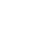represent a transformation T, triangle ABC where A(1,1) B(5,1) and C(2,4) is mapped onto A1B1C1 by T.
1.
1. Find the coordinates of the image A1B1C1 of ABC under T. (2 marks)
2. On the grid provided draw ABC and its image A1B1C1 (2 marks)3. Describe the transformation T (1 mark)
2. Draw A2B2C2 image A1B1C1 under an enlargement center (0, 0) scale factor - 1/2 . (2 marks)
3. Find a single matrix that would A2B2C2 onto ABC. (3 marks)
5. The probability that Hilda, Lucy and Caroline will be late for breakfast on any one morning are 1/4 , 1/3 and 1/5 respectively.
1. Using a probability tree diagram find the probability that:-
1. None of them will be late (2 marks)
2. Only one of them will be late (3 marks)
3. At least one of them will be late (3 marks)
4. At most one of them will be late (2 marks)
6. The figure below represents a square based pyramid with equilateral triangles AB=5cmCalculate the
1. Height of the triangular faces (2 marks)
2. Length of AC (1 mark)
3. Angle between VA and ABCD (2 marks)
4. Angle between VAD and ABCD (2 marks)
5. Angle between VAB and VCD (3 marks)
7. A triangular plot ABC is such that AB = 36m, BC = 40cm and AC = 42m
1. Calculate
1. Area of the plot in square metres (3 marks)
2. Acute angle between the edges AB and BC (2 marks)
2. A circular fence passes through vertices A, B and C. A water tap is to be installed inside the plot such that the tap is equidistant from each of the vertices A, B and C. Calculate
1. The distance of the tap from vertex A (2 marks)
2. The area between the circular fence and the triangular plot (3 marks)
8. Fill the table below for the function y=x3+4x2-x-6 for-5≤x≤3. (2 marks)
 X -5 -4 -3 -2 -1 0 1 2 3 Y -26
1. On the grid provided draw the graph of y=x3+4x2-x-6 for-5≤x≤3.Use the scale of 1cm to represent 1 unit horizontally and 1cm to represent 10 units vertically. (3 marks)2. Use your graph to solve the following;
1. x3+4x2-x-6=0 (2 marks)
2. 3x3+12x2-15x-21=0 (3 marks)## MARKING SCHEME

SECTION I (50 Mks)
(Answer all questions in this section)

1. Use logarithms tables to evaluate. (4 marks)
 No Log 130.9 2.1169 27.68 1.4422 + 100.9 2.0039 3.4461 2.6708
2.6708 x 2/3
3.3416
3    3
1.1139
antilog = 0.1300
all 3 logs - m1
attempt to add and subtrsct - m1
multiplying and dividing - m1
accuracy - a1
2. A trader mixes grade A coffee costing sh 600 per kg, with grade B coffee costing sh. 280 per kg in the ratio 3 : 5.Find the price at which he must sell 1 kg of the mixture to make a profit of 20 % . (4 marks)
Cost
[3/8 x 600] + [5/8 x 280]
225 + 175
= 400
120/100 x 400
= sh 480
3. Given that cos θ =, find the value ofin its simplest form. (Leave your answer in surd form) (3 marks)4. Determine the equation of the normal to the curve y = 3x2 - 4x + 5 at the point (1, 4). (3 marks)
y = 3x2 - 4x + 5
dy/dx = 6x - 4
(1, 4)
dy/dx = (6 x 1) - 4
= 2
2 x gN = -1
gH = -1/2
y - 4 = -1
x - 1    2
y = -1/2x + 1/2 + 4
y = -1/2x + 41/2
5. Water flows from a pipe at the rate of 250 litres per minute. If the pipe is used to drain a tank full of water measuring 3.2m by 2.5m by 2m, how long would it take to drain the tank completely when it is 3/4 full? (3 marks)
Volume = [3.2 x 2.5 x 2] x 3/4
= 16 x 3/4 = 12m3
volume = 12 x 1000
=12000L
Time = 12000
250
= 48 minutes
6. Make N the subject of the formula(2 marks)
t x (3N - p) = 5p - H x (3N - p)
3N - p
multiplying both sides by (3N - p)
3Nt - tp = 5P - N
3Nt + N = 5p + tp
N(3t + 1) = 5p + tp
N = 5p + tp
3t + 1
7. Determine the period and amplitude of the function. y = 4 sin (2x - 20°) (2 marks)
Amplitude = 4
Period = 360 = 180º
2
8. In the figure below, PA is 1.2cm shorter than PD. Given that AB = 15.6cm, CD = 9.6cm,Determine the length of PA. (3 marks)
[15.6 + x]x = [x + 1.2 + 9.6][x + 1.2]
15.6x + x2 = [x + 10.8][x + 1.2]
15.6x + x2 = x2 + 12x + 12.96
x = 12.96
3.6
= 3.6 cm
9. Without using logarithms table or calculator, solve for x in;
log 5 – 2 + log(2x + 10) = log (x - 4) (3 marks)
log 5  + log(2x + 10) - log(x  4) = 2
log 5 (2x + 10)= 2
x - 4
102 = 5(2x + 10)
x - 4
x = 5
10. In an arithmetic progression, the 20th term is 92 and the sum of the first 20 terms is 890. Calculate;
1. The first term (2 marks)
a + 19d = 92
20/2 {2a + 19d} = 890
a + 19d = 92
2a + 19d = 89
-a = 3
a = -3
2. The common difference (1 mark)
a + 19d = 92
d = 92 + 3
19
d = 5
11. Solve for θ in the equation sin (3θ + 120º) =for 0 ≤ θ ≤ 180º. (3 marks)
3θ + 120 = sin-1 √3/2 = 60º (B1 for 60º)
3θ + 120 = 60º, 120º, 420º, 480º, 780º (m1 for first four)
θ = -20º, 0º, 100º, 120º, 220º
∴ θ = 0º, 100º, 120º (A1 for all 3)
12.
1. Expand and simplify the expressionup to the third term. (2 marks)
1(4x)5 . (-y/2)º + 5(4x)4(-y/2)1 + 10(4x)3(-y/2)2
(1 × 1024x5 x × 1) +  (5 × 256 × x4 × -y/2) + [10 × 64 × x3.y2/4)
1024x5 - 640x4y + 160x3y2
2. Hence use the expansion in (a) above to approximate the value of (39.6)5 (2 marks)
39.6 = (40 - 0.4)
∴ 4x = 40  &  y/2 = 0.4
x = 10            y = 0.8
(1024
× 105) - 640 × 104 × 0.8 + 160 × 103 × 0.82
= 97,382, 400
13. The cost per head for catering for a party is partly constant and partly varies inversely as the number of people expected. The cost per head for a party of 100 people is Sh. 1860 and that for 180 people is sh. 1060. Find the cost per head for 200 people. (3 marks)
c α k + 1/n
c = k  + m/n
k \$ m constant
1860 = k + m/100
1060 = k + m/180
186000 = 100k + m
190800 = 180k + m
-4800 = - 80k
k = 60

m = 186000 - 100 x 60
=180 000
c = 60 + 180000
n
c = 60 + 180,000
200
= 960
14. A body is moving along a straight line and its acceleration after t seconds is (5 – 2t) ms-2. Its initial velocity Vms-1 is 4ms-1. Find V in terms of t. (3 marks)
v = ∫ (5 - 2t) dt
v = 5t - t2 + c
4 = (5
× 0) - 02 + c
c = 4
v = 5t - t2 + 4
15. Determine the turning points for the curve y = 5x – 8x2 + x3. (4 marks)
dy/dx = 5 - 16x + 3x2
3x2 - 16x + 5 = 0
(3x - 1) (x - 5) = 0
x = 1/3 & x = 5
(for both)
when x = 1/3
y = (5
× 1/3) - 8 × (1/3) + (1/3)
y = 22/27
(1/3, 22/77)
when x = 5
y = -50
(1/3 , 22/27) (5, -50)
16. Draw a line PQ = 7.2cm and on one side of the line, use a ruler and pair of compasses only to draw the locus of a point A such that ∠ PAQ = 60º and on it mark point A such that PA = QA (3 marks)SECTION II (50 Mks)
(Answer any FIVE questions from this section)

1. The table below represents marks scored in a mathematics test.
 Marks 10-19 20-29 30-39 40-49 50-59 60-69 70-79 No. of students 2 6 7 13 6 4 2
Using an assumed mean of 44.5, Determine
1. Mean marks for the test (3 marks)
 class x f t = x - A f.t t2 ft2 c.f 10-19 14.5 2 -30 -60 900 1800 2 20-29 24.5 6 -20 -120 400 2400 8 30-39 34.5 7 -10 -70 100 700 15 40-49 44.5 13 0 0 0 0 50-59 54.5 6 10 60 100 600 60-69 64.5 4 20 80 400 1600 70-79 74.5 2 30 60 900 1800 ∑f = 40 ∑ft = -50 ∑ft2 = 8900
column of ft
t = ∑ft = - 50
∑p      40
t = -1.25
x = 44.5 - 1.25
=43.25
2. Standard deviation (4 marks)
column of ft2
∑ft2 = 8900
√8900/40 - (-1.25)2
ans = 14.86
3. Determine the pass mark if 30% of the students failed the exam. (3 marks)
30/100 x 40 =12 failed
pass mark => 13th std
c.f up to 3rd class
29.5 + (13 - 8)10
7
29.5 + 7.143
=36.64
2.
1. Draw the curve of the function y = 18 + 3x – x2 for -3 ≤ x ≤ 5. (3 marks)
Use a scale of 2cm to represent 1 unit on x axis and 1cm to represent 2 unit on y axis.
 x -3 -2 -1 0 1 2 3 4 5 y 0 8 14 18 20 20 18 14 182. Find the actual area bounded by the curve, the x-axis and the line X= 5. (2 marks)3. By using trapezoidal rule with five ordinates, Estimate the area bounded by the curve, the x-axis and the line X= 5. (3 marks)4. Find the percentage error introduced by the approximation. (2 marks)
1171/3 - 112 x 100
1171/3
= 4.545%
3. An airplane leaves town A (83°N, 155°W) to town B (40°N, 25°E) using the shortest route at a speed of 450 knots. (Take π = 22/7 and radius of the earth R = 6370km).
1.
1. Calculate the distance between A and B in nautical miles. (2 marks)155 + 25 = 180º
Thro North pole
θ = 180 - (83 + 40) = 57º
60 x 57 = 3420 nm
2. Calculate the time taken to travel from town A to B (2 marks)
time = 3420
450
= 7.6 hours or 7 hours 36 mins
2. From B, the plane flies westwards along the latitude to town C (40°N, 13°W). Calculate the distance BC in kilometers. (3 marks)
(40ºN, 25ºE) (40ºN, 13ºK)
α = 25 + 15 = 40º
40/360 x 2 22/7 x 6370 cos 40
= 3408.05 km
3. From town C, the plane took off at 3:10 pm towards town D (10°N, 13°W), at the same speed. At what time did the plane land at D? (3 marks)40 - 10 = 30º
60 x 30 = 1800nm
time = 1800 = 4 hours
450
3.10
4
7.10 pm
4. The matrixrepresent a transformation T, triangle ABC where A(1,1) B(5,1) and C(2,4) is mapped onto A1B1C1 by T.
1.
1. Find the coordinates of the image A1B1C1 of ABC under T. (2 marks)2. On the grid provided draw ABC and its image A1B1C1 (2 marks)3. Describe the transformation T (1 mark)
A shear x-axis invariant and c(2, 4) -------> c1
2. Draw A2B2C2 image A1B1C1 under an enlargement center (0, 0) scale factor - 1/2 . (2 marks)3. Find a single matrix that would A2B2C2 onto ABC. (3 marks)
5. The probability that Hilda, Lucy and Caroline will be late for breakfast on any one morning are 1/4 , 1/3 and 1/5 respectively.
1. Using a probability tree diagram find the probability that:-
1. None of them will be late (2 marks)2. Only one of them will be late (3 marks)3. At least one of them will be late (3 marks)4. At most one of them will be late (2 marks)6. The figure below represents a square based pyramid with equilateral triangles AB=5cmCalculate the
1. Height of the triangular faces (2 marks)
k = √52 - 2.52
= 4.330 cm
2. Length of AC (1 mark)
= √52 + 52
= 7.071 cm
3. Angle between VA and ABCD (2 marks)
cos θ = 3.536
5
θ = cos-1  3.536
5
θ = 45º
4. Angle between VAD and ABCD (2 marks)
cos θ = 2.5
4.33
θ cos -1  2.5
4.33
θ = 54.73º
5. Angle between VAB and VCD (3 marks)180 - (90 + 54.73)
= 35.27
35.  27 x 2
= 70.54
7. A triangular plot ABC is such that AB = 36m, BC = 40cm and AC = 42m
1. Calculate
1. Area of the plot in square metres (3 marks)2. Acute angle between the edges AB and BC (2 marks)
1/2 x 36 x 40 sin B = 662.05
sin B = 0.9195
B = sin-1 0.9195
= 66.85º
2. A circular fence passes through vertices A, B and C. A water tap is to be installed inside the plot such that the tap is equidistant from each of the vertices A, B and C. Calculate
1. The distance of the tap from vertex A (2 marks)2R   =      42
sin 66.85º
R = 22.84m
2. The area between the circular fence and the triangular plot (3 marks)
πr2 - 662.05
22/7 x 22.842 = 1639.52
A = 1639.52 - 662.05
A = 977.47m2
8. Fill the table below for the function y=x3+4x2-x-6 for-5≤x≤3. (2 marks)
 X -5 -4 -3 -2 -1 0 1 2 3 Y -26 -2 6 4 -2 -6 -2 16 54
1. On the grid provided draw the graph of y=x3+4x2-x-6 for-5≤x≤3.Use the scale of 1cm to represent 1 unit horizontally and 1cm to represent 10 units vertically. (3 marks)2. Use your graph to solve the following;
1. x3+4x2-x-6=0 (2 marks)
y = x3 + 4x2 - x - 6
0 = x3 + 4x2 - x - 6
y = 0
x = -3.8, x = -1.3 & 1.1
2. 3x3+12x2-15x-21=0 (3 marks)
y = x3 + 4x2 - x - 6
0 = x3 + 4x2 - 5x - 7
y = 4x + 1
x = -4.7, -1, 1.6

• ✔ To read offline at any time.
• ✔ To Print at your convenience
• ✔ Share Easily with Friends / Students